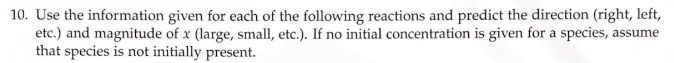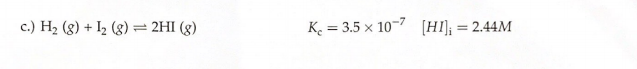# 10. Use the information given for each of the following reactions and predict the direction (right, left, etc.) and magnitude of x (large, small, etc). If no initial concentration is given for a species, assume that species is not initially present. c.) H2 (g) I2 (g) ⇌ 2HI (g) Kc = 3.5 X 10^-7 [HI]i = 2.44M